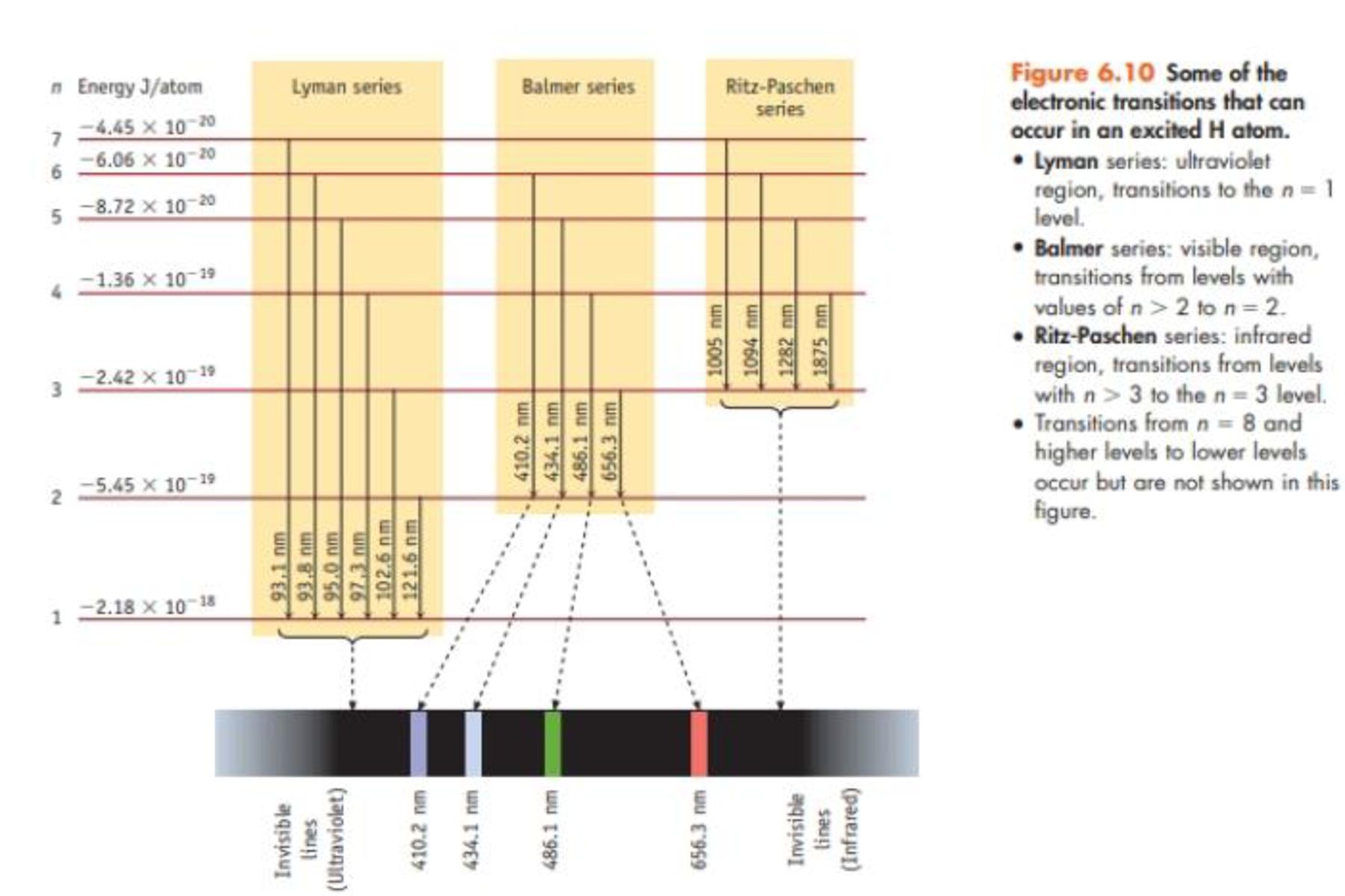Chapter 6, Problem 81SCQ

Chapter
Section
Textbook Problem

A photon with a wavelength of 93.8 nm strikes a hydrogen atom, and light is emitted by the atom. How many emission lines would be observed? At what wavelengths? Explain briefly (see Figure 6.10).Interpretation Introduction

Interpretation The total number of emission lines and its corresponding wavelength of an atom which emit light at 93.8nm have to be explained briefly.

Concept introduction:

• Electronic transitions that take place in excited H atom is,
1. a. Lyman series: electronic transitions take place to the n=1 level and it is in ultraviolet region.
1. b. Balmer series: electronic transitions take place from n>2 to the n=2 level and it is in visible region.
2. c. Ritz-Paschen series: electronic transitions take place from n>3 to the n=3 level and it is in infrared region.
3. d. Brackett series: electronic transitions take place from n>4 to the n=4 level.
4. e. Pfund series: electronic transitions take place from n>5 to the n=5 level
• The frequency of the light is inversely proportional to its wavelength.

ν=cλwhere, c=speedoflightν=frequencyλ=wavelength

Explanation

The photon has a wavelength of 93.8nm which implies that in this transition, the photons are emitted from n=6.

Hence, the highest electronic state is n=6 so the electronic transition from n=6,5,4,3,2 is possible to each of the corresponding lower electronic states.

Therefore the total number of emission lines is 15, if only six quantum levels are considered. Each of the emission line of the photon corresponds to a different wavelength.

• The wavelength of emission line corresponding to transition of photons from n=6,5,4,3,2 to the n=3 electronic states.

The wavelength of emission line corresponding to transition of photons from n=6 to n=3 level is at 1094nm. The wavelength of emission line corresponding to transition of photons from n=5 to n=3 level is at 1282nm. The wavelength of emission line corresponding to transition of photons from n=4 to n=3 level is at 1875nm.

• The wavelength of emission line corresponding to transition of photons from n=6,5,4,3,2 to the n=2 electronic states.

The wavelength of emission line corresponding to transition of photons from n=6 to n=2 level is at 410

Still sussing out bartleby?

Check out a sample textbook solution.

See a sample solution

The Solution to Your Study Problems

Bartleby provides explanations to thousands of textbook problems written by our experts, many with advanced degrees!

Get Started

Find more solutions based on key concepts